### Home > AC > Chapter 9 > Lesson 9.2.2 > Problem9-44

9-44.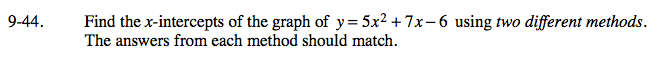To find the x-intercepts, make y = 0.

Method 1:

Draw a generic rectangle and diamond.

Set y = 0.

0 = 5x² + 7x − 6

The missing numbers in the square should equal 7x.

0 = (x + 2)(5x − 3)

x + 2 = 0 or 5x − 3 = 0

x = −2

$x=\frac{3}{5}$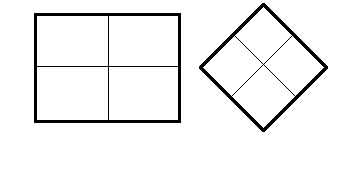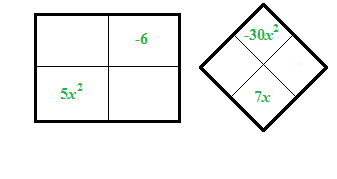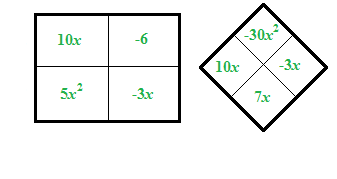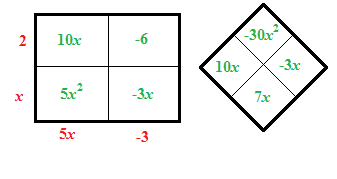Method 2:

Substitute the a, b, and c values of the expression into the quadratic formula.

Finish the rest yourself and show that both methods give the same answer.

$x= \frac{-b±\sqrt{b^2 - 4ac}}{2a}$

$x= \frac{-7±\sqrt{7^2 - 4(5)(-6)}}{(2)(5)}$

$x= \frac{-7±\sqrt{49 + 120}}{10}$